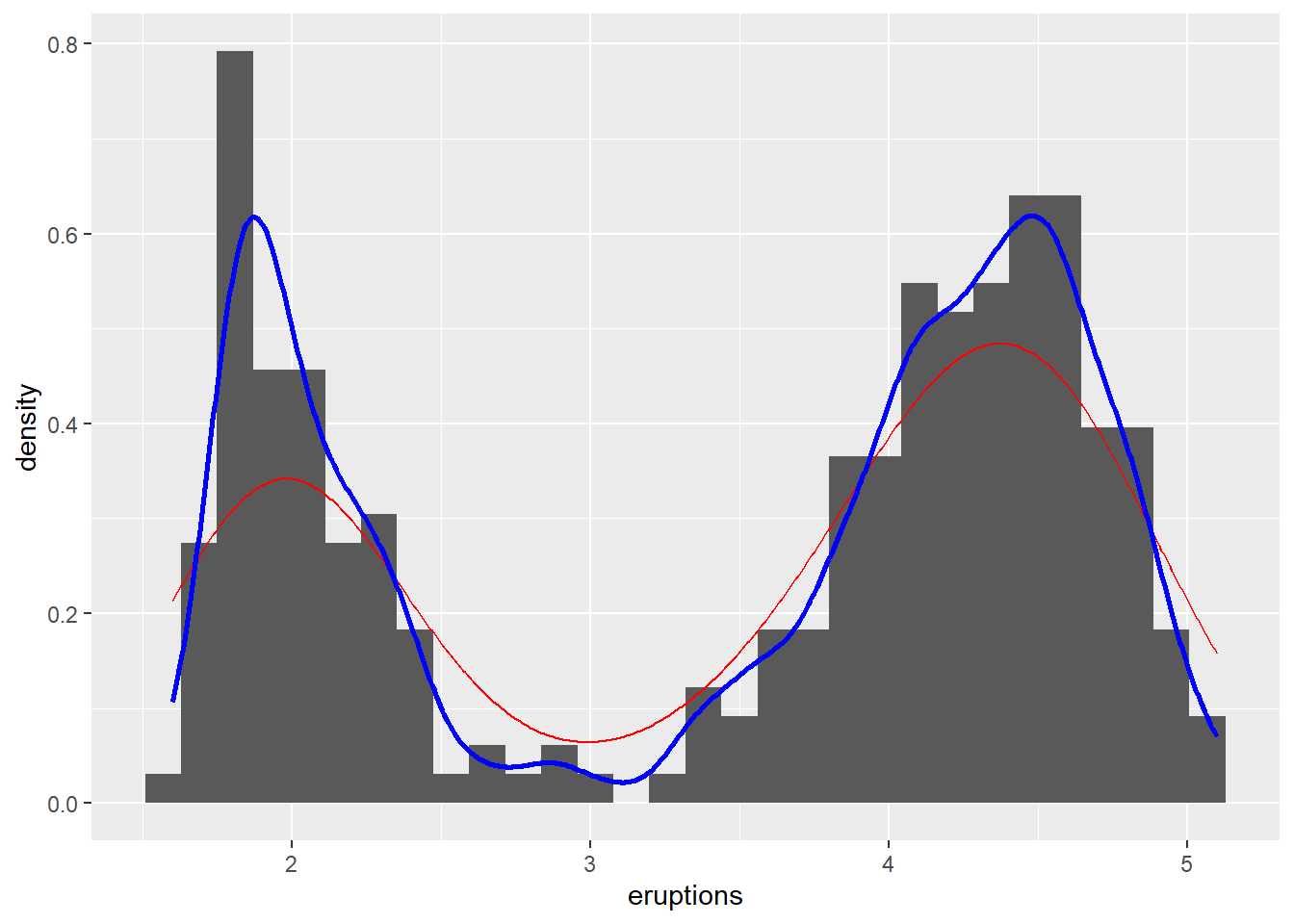## Overview

This notebook compliments the Nonparametrics lecture slides.

### How to use an Rnotebook

This is an R Markdown Notebook. When you execute code within the notebook, the results appear beneath the code.

Try executing this chunk by clicking the Run button within the chunk or by placing your cursor inside it and pressing Cmd+Shift+Enter.

Add a new chunk by clicking the Insert Chunk button on the toolbar or by pressing Cmd+Option+I.

When you save the notebook, an HTML file containing the code and output will be saved alongside it (click the Preview button or press Cmd+Shift+K to preview the HTML file).

The preview shows you a rendered HTML copy of the contents of the editor. Consequently, unlike Knit, Preview does not run any R code chunks. Instead, the output of the chunk when it was last run in the editor is displayed.

## Examples: Old Faithful

To illustrate some points about density estimation, we’ll use data the Old Faithful Geyser data set from the mass package. This data contains waiting times between eruptions and the duration of the eruption for the Old Faithful geyser in Yellowstone National Park, Wyoming, USA. For more info ?faithful.

First, load and plot the data using this base hist() function.

# KDENSITY EXAMPLE
library(MASS)
library(ggplot2)

hist(faithful$waiting)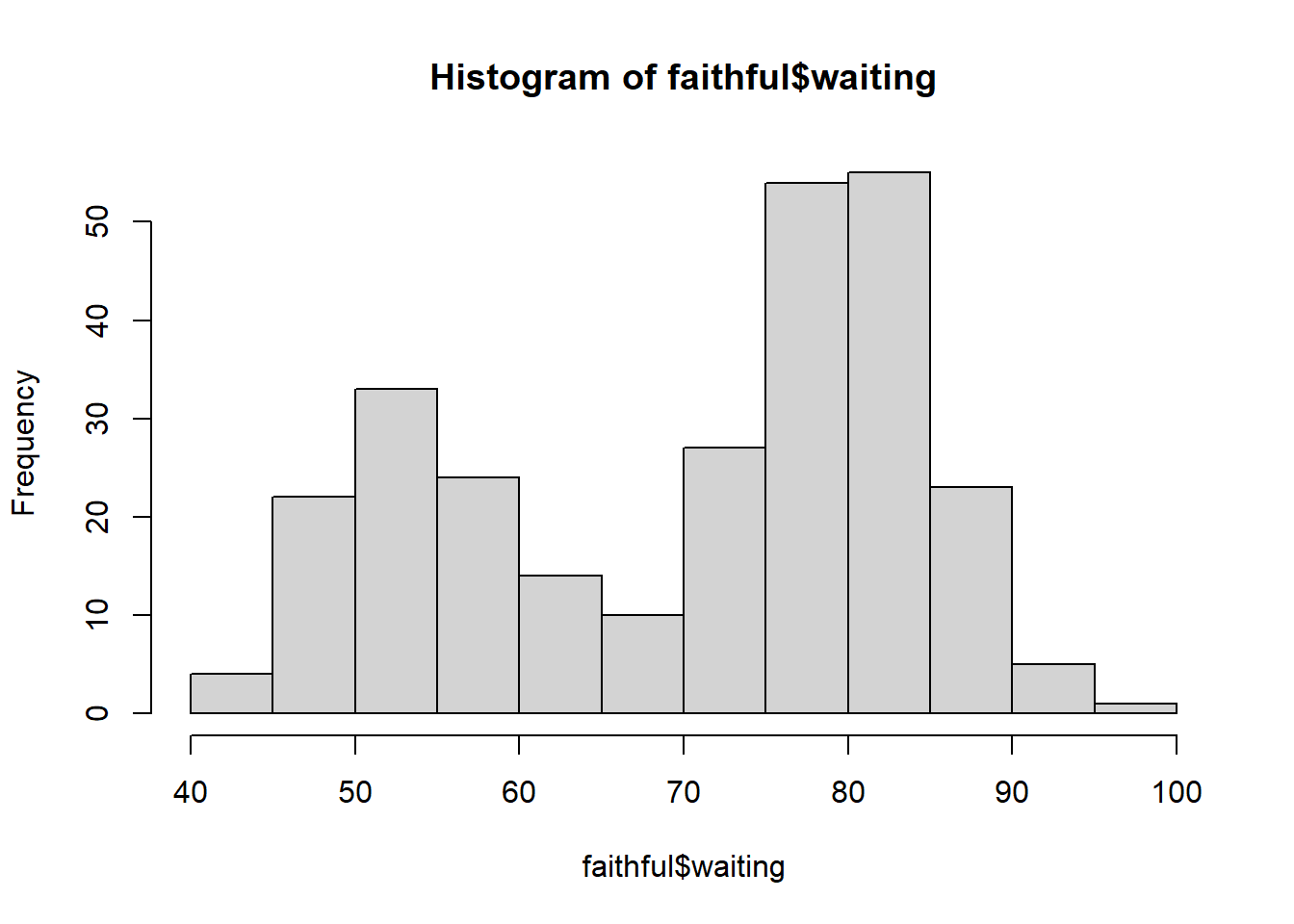We see that there are two modes (not going to guess why), and around each things aren’t obviously symmetric. To highlight the relationship between bias and variance in a histogram, lets set the bins to 2 and 25. par(mfrow=c(1,2)) hist(faithful$waiting,1, main = "bins = 2")
hist(faithful$waiting,25, main = "bins = 25")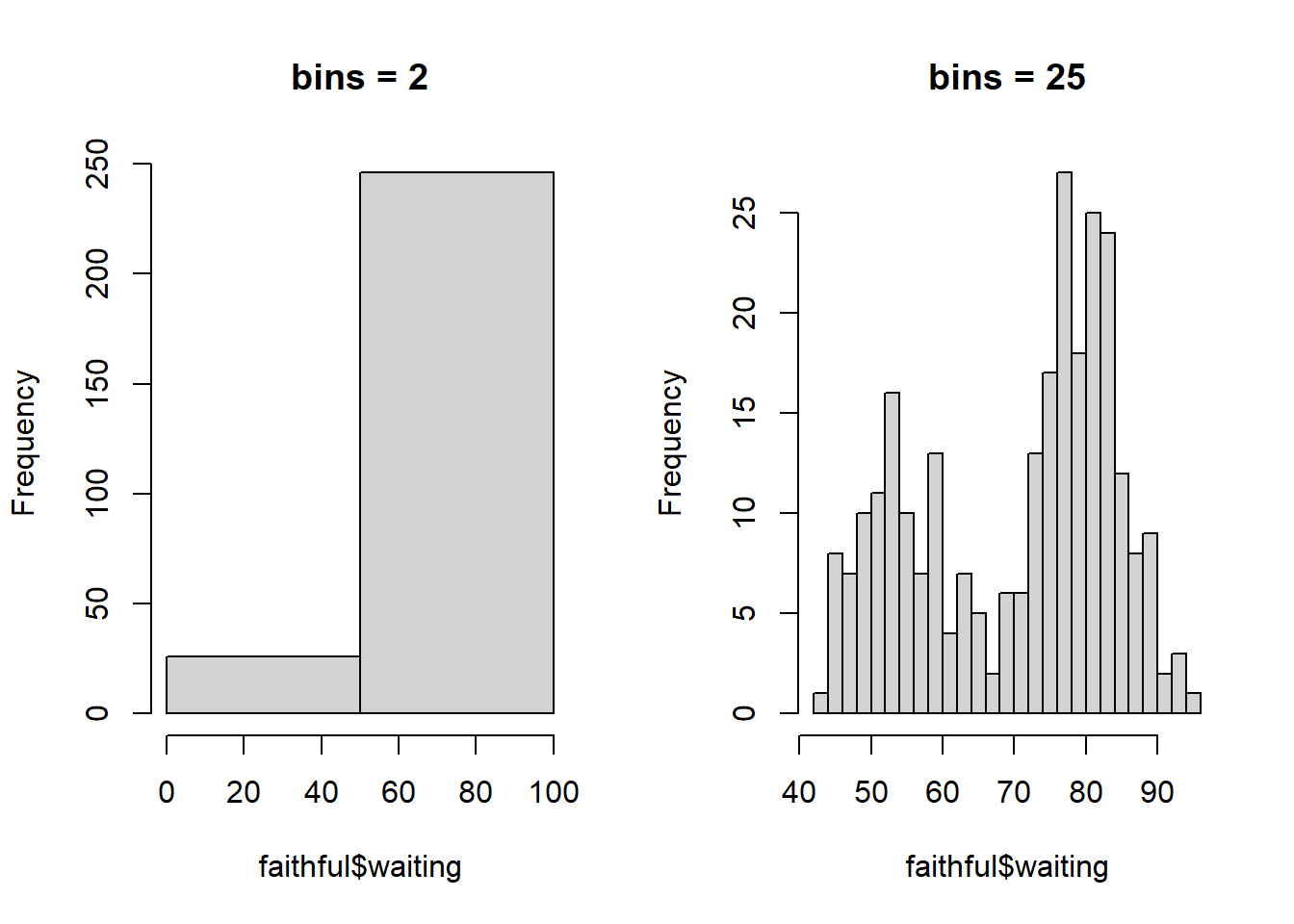There are now many density estimation packages. But the base density function in the stats package is sufficient for now. Lets look at the available kernels. (kernels <- eval(formals(density.default)$kernel))
##  "gaussian"     "epanechnikov" "rectangular"  "triangular"   "biweight"
##  "cosine"       "optcosine"
## show the kernels in the R parametrization
plot (density(0, bw = 1), xlab = "",
main = "R's density() kernels with bw = 1")
for(i in 2:length(kernels))
lines(density(0, bw = 1, kernel =  kernels[i]), col = i)
legend(1.5,.4, legend = kernels, col = seq(kernels),
lty = 1, cex = .8, y.intersp = 1)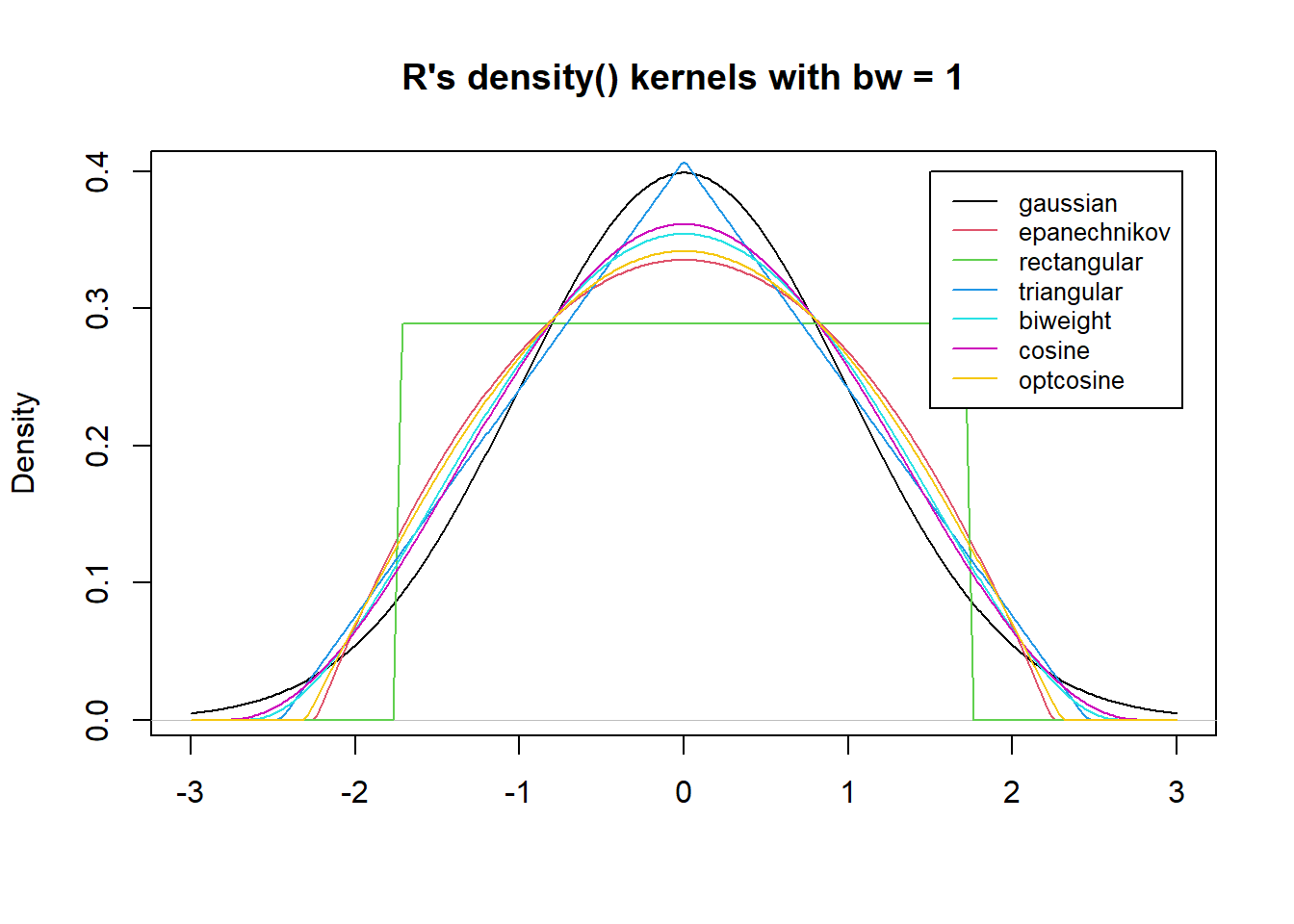How much does this matter for the Old Faithful data? Let’s consider three common ones.

par(mfrow=c(1,3))

plot(density(faithful$waiting,bw=2, kernel = c("rectangular")), main = "rectangular") plot(density(faithful$waiting,bw=2, kernel = c("gaussian")),
main = "gausian")

plot(density(faithful$waiting,bw=2, kernel = c("epanechnikov")), main = "epanechnikov")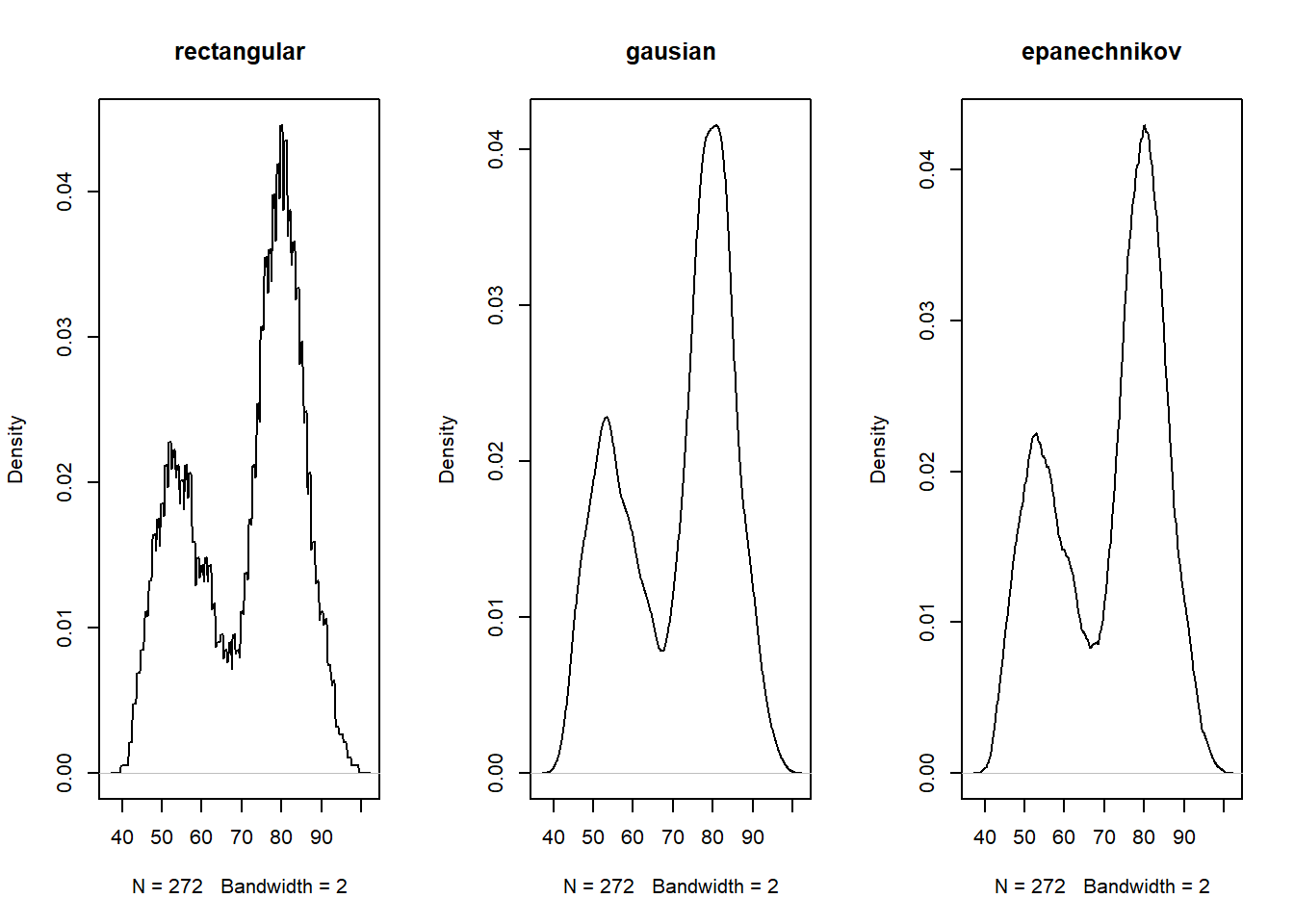## What is the optimal bandwidth? For a Gaussian kernel, it is common to use Silverman’s plug-in estimate for the optimal bandwidth, $$h^*_n=0.9*\min(s,IQ/1.34)*n^{-1/5}$$, where we replace $$s$$ is the sample standard deviation. You can easily calculate this, however the stats package will give you this directly by via bw.nrd0. You can find a description of the other built in bw options here. eruptions = faithful$eruptions
n = length(eruptions)
iq = IQR(eruptions)
(bw_plugin = .9*min(sd(eruptions),iq/1.34)*n^(-1/5))
##  0.334777
bw.nrd0(eruptions)
##  0.334777

Alternatively, we can use cross-validation to search for the optimal bandwidth.

set.seed(1)

X = eruptions

J<- function(h){
fhat=Vectorize(function(x) density(X,from=x,to=x,n=1,bw=h)$y) fhati=Vectorize(function(i) density(X[-i],from=X[i],to=X[i],n=1,bw=h)$y)
F=fhati(1:length(X))
return(integrate(function(x) fhat(x)^2,-Inf,Inf)$value-2*mean(F)) } vx=seq(.05,.2,by=.01) vy=Vectorize(J)(vx) df=data.frame(vx,vy) qplot(vx,vy,geom="line",data=df)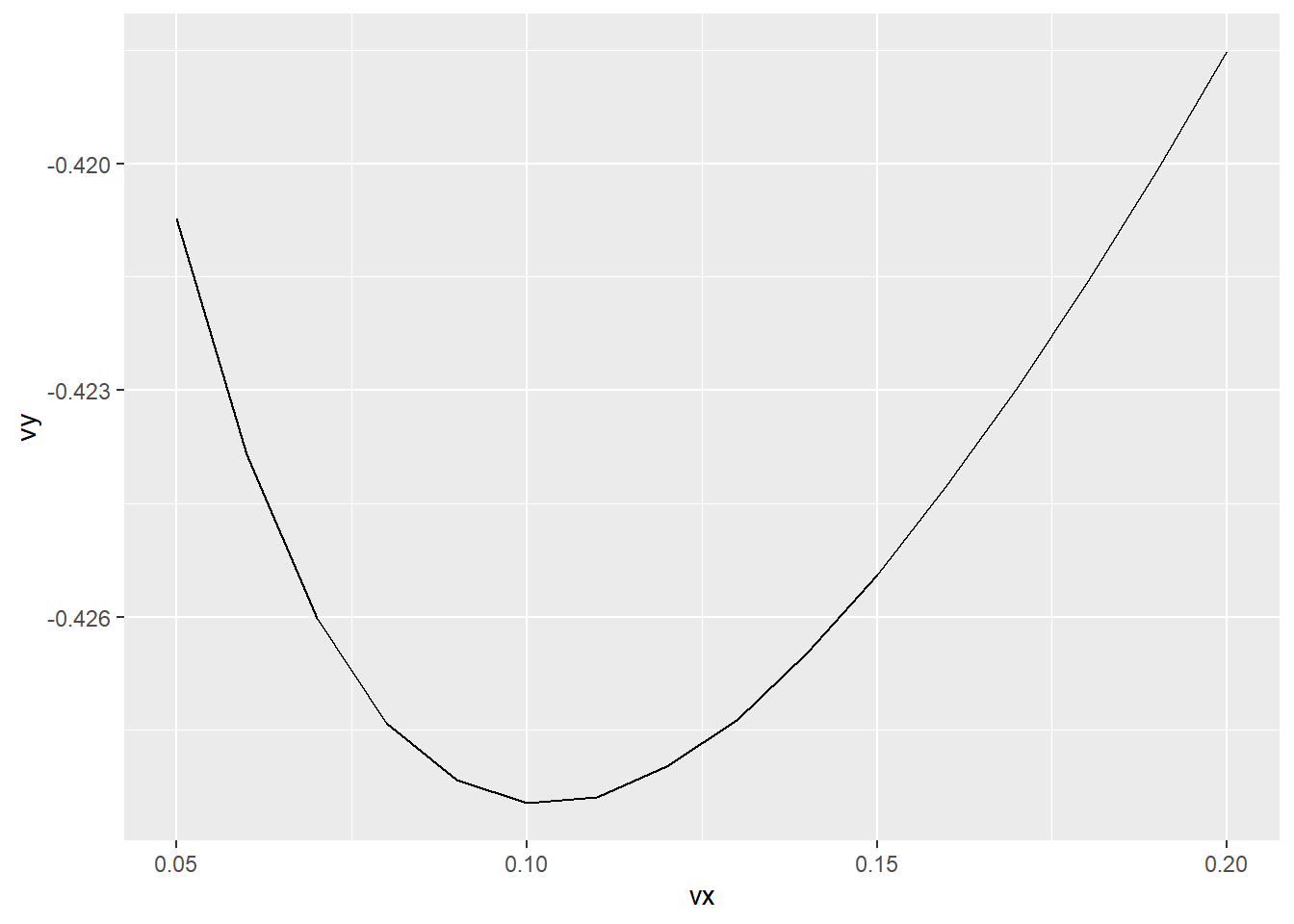(myopt <- optimize(J,interval=c(.01,.8)) ) ##$minimum
##  0.1042313
##
## $objective ##  -0.4284783 bw_cv <- myopt$minimum

## note that stats actually has a builtin cv method as well
bw.ucv(eruptions)
##  0.1019193

### Compare density plots

Here the plugin bindwith is more than three times larger than the cross-validation binwidth. Let’s plot what that difference looks like.

# PLOT
data <- as.data.frame(eruptions)

ggplot(data,aes(eruptions))  + geom_histogram(aes(y = stat(density))) +
geom_line(stat="density",bw=bw_plugin, col = 'red') +
geom_line(stat="density", bw=bw_cv,lwd = 1, col = 'blue') 
## stat_bin() using bins = 30. Pick better value with binwidth.